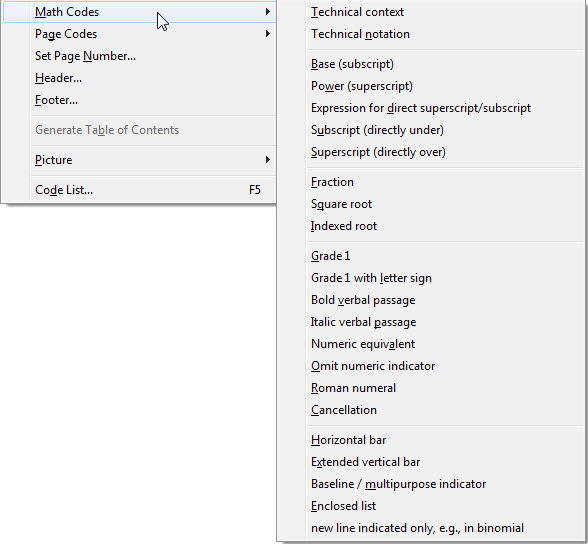### Layout: Math CodesThis menu contains the DBT codes related to translating and formatting mathematics.

Examples of these codes are given in the How-To Guide, a DBT document which is typically installed as and found in C:\Program Files\Duxbury\DBT 12.5\Documentation (English)\dochow.dxp. (On 64 bit systems, look in "Program Files (X86)".)

Unless you are an experienced math braillist, instead of entering math codes directly into DBT you may prefer using a mathematics editor program such as Scientific Notebook or MathType to prepare your material, and then import the resultant file.

Scientific Notebook is a popular program for producing printed mathematical books, papers, exercises, exam papers, etc. Files produced by this program can be imported into DBT, and converted into Mathematical Braille. Note that currently only English (American, Nemeth and British) and French Mathematical Braille are supported.

MathType is an add-on program for Microsoft Word which facilitates placing mathematical formulae into Word documents. These documents containing MathType equations can be opened in DBT, which will translate them into mathematical braille.

For more details consult the topics Scientific Notebook or MathType.

If you want to work in DBT directly, without the assistance of a math editor, here is a basic guide to inserting math codes directly into DBT.